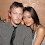## Wednesday, January 27, 2016

### 33 39 47 66 96 119 | The Death of actor Abe Vigoda

Godfather = 7+6+4+6+1+2+8+5+9 = 48
Godfather = 7+15+4+6+1+20+8+5+18 = 84

Barney = 2+1+9+5+5+7 = 29
Miller = 4+9+3+3+5+9 = 33
Barney Miller = 62 (Mason)

Barney = 2+1+18+14+5+25 = 65
Miller = 13+9+12+12+5+18 = 69
Barney Miller = 134

Abe is best remembered as a Jew who played an italian  gangster in the Godfather films.

Abraham = 1+2+9+1+8+1+4 = 26 (Abe = 1+2+5 = 8)
Charles = 3+8+1+9+3+5+1 = 30/39
Vigoda = 4+9+7+6+4+1 = 31
Abraham Charles Vigoda = 87/96 (Freemason = 96)
Abe Vigoda = 39

Abraham = 1+2+18+1+8+1+13 = 44 (Abe = 1+2+5 = 8)
Charles = 3+8+1+18+12+5+19 = 66
Vigoda = 22+9+7+15+4+1 = 58
Abraham Charles Vigoda = 168
Abe Vigoda = 66

2/24/1921 = 2+24+19+21 = 66 (Abe Vigoda) (Charles)
2/24/1921 = 2+24+(1+9+2+1) = 39 (Abe Vigoda)
2/24/1921 = 2+2+4+1+9+2+1 = 21
2/24/21 = 2+24+21 = 47

1/26/2016 = 1+26+20+16 = 63
1/26/2016 = 1+26+(2+0+1+6) = 36
1/26/2016 = 1+2+6+2+0+1+6 = 18
1/26/16 = 1+26+16 = 43

Notice there is no connection between his birth and death numerology.  I suspect this is a natural passing.

Phil = 7+8+9+3 = 27
Fish = 6+9+1+8 = 24/33
Phil Fish = 51/60

Phil = 16+8+9+12 = 45
Fish = 6+9+19+8 = 42
Phil Fish = 87

Sal = 1+1+3 = 5/14
Tessio = 2+5+1+1+9+6 = 24/42
Sal Tessio = 29/38/56

Sal = 19+1+12 = 32
Tessio = 20+5+19+19+9+15 = 87
Sal Tessio = 119

Nice 3:03 tweet time, for 'Good Burger' remembrance.

Good = 7+6+6+4 = 23
Burger = 2+3+9+7+5+9 = 35
Good Burger = 58 (Freemasonry)

Good = 7+15+15+4 = 41
Burger = 2+21+18+7+5+18 = 71
Good Burger = 112

1.1.2.